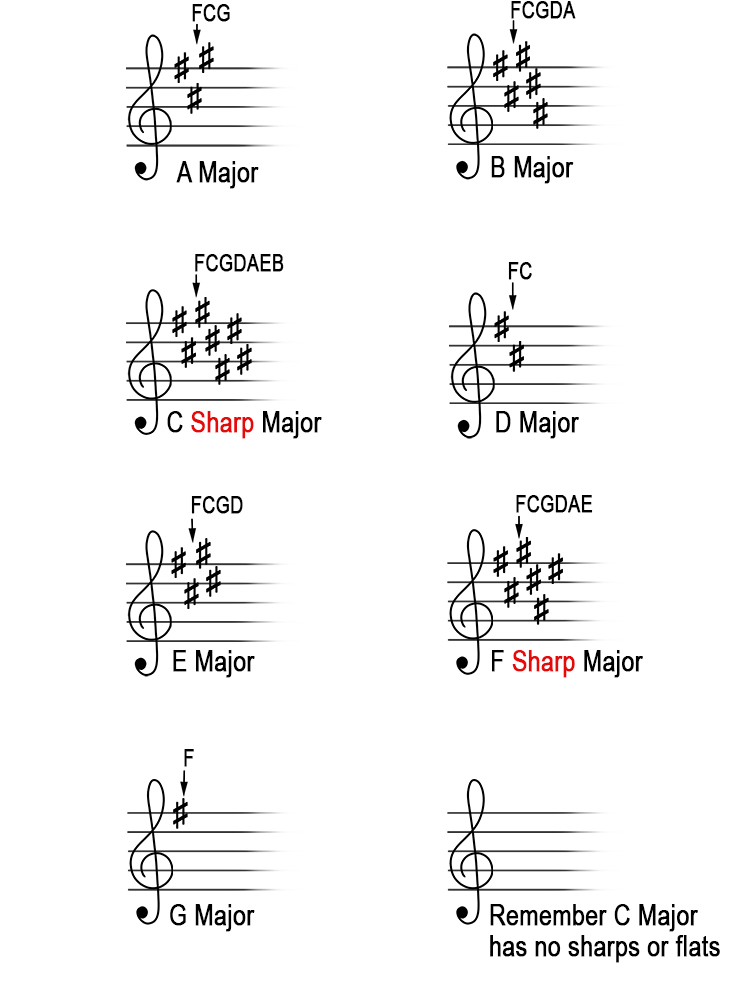# Given the major key, what are the sharps? Method 1

The order of the sharps are always
F C G D A E B

The above is recalled by remembering: 'Fat Cats Go Down Alleys Eating Bacon'.

We know the order of the sharps but if you are asked in an exam what are the sharps in D major, this is how you would work it out:

TAKE OFF ONE ALPHABET LETTER
AND USE ALL THE SHARPS UP TO AND INCLUDING THAT LETTER IN THE ORDER OF THE SHARPS KEY SIGNATURE

If the major sharp key listed is:

A major then it's G.           Sharps = F C G

B major then it's A.           Sharps = F C G D A

C sharp major then it's B. Sharps = F C G D A E B

D major then it's C.           Sharps = F C

E major then it's D.           Sharps = F C G D

F sharp major then it's E.  Sharps = F C G D A E

G major then it's F.           Sharps = F